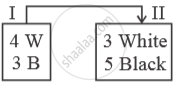Advertisement Remove all ads

# Bag a Contains 4 White Balls and 3 Black Balls. While Bag B Contains 3 White Balls and 5 Black Balls. Two Balls Are Drawn from Bag a and Placed in Bag B. Then, What is the Probability of - Mathematics

Sum

Bag A contains 4 white balls and 3 black balls. While Bag B contains 3 white balls and 5 black balls. Two balls are drawn from Bag A and placed in Bag B. Then, what is the probability of drawing a white ball from Bag B?

Advertisement Remove all ads

#### SolutionCase I : Let Both white balls are transfered from bag I to bag II and then a white ball is drawn from bag II.

Required probability = (""^4C_2) / (""^7C_2) × (""^5C_1) / (""^10C_1)

= (4xx3)/(7xx6)xx5/10

=2/7xx1/2=1/7

Case II: Let both black balls are transfered from bag I to bag II and then a white ball is drawn from bag II.

Required probability = (""^3C_2) / (""^7C_2) × (""^3C_1) / (""^10C_1)

= (3xx2)/(7xx6) xx 3/10

= 1/7xx3/10=3/70

Case III: 1 white and 1 black ball is transfered and then a white ball is drawn from bag II.

Required probability = (""^4C_1xx""^3C_1)/(""^7C_2) xx (""^4C_1)/(""^10C_1)

=(4xx3)/(7xx3)xx4/10

= 16/70
Total probability = Case I + Case II + Case III
= 1/7 + 3/70 + 16/70

= 10/70+ 19/70

= 29/70

Is there an error in this question or solution?
Advertisement Remove all ads

#### APPEARS IN

Advertisement Remove all ads

#### Video TutorialsVIEW ALL 

Advertisement Remove all ads
Share
Notifications

View all notifications

Forgot password?